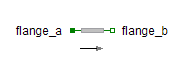Multiphase Variable Capacitor - MapleSim Help

Multiphase Variable Capacitor

Ideal linear electrical capacitors with variable capacitanceDescription The Multiphase Variable Capacitor (or Variable Capacitor) component contains $m$ single-phase variable capacitor models, each connected between corresponding phases of ${\mathrm{plug}}_{p}$ and ${\mathrm{plug}}_{n}$, with the capacitance controlled by the corresponding signal of an input vector.Connections

 Name Description Modelica ID ${\mathrm{plug}}_{p}$ Positive $m$-phase plug plug_p ${\mathrm{plug}}_{n}$ Negative $m$-phase plug plug_n $C$ Real input $m$-vector; capacitance in $F$ CParameters

 Name Default Units Description Modelica ID $m$ $3$ Number of phases m ${C}_{\mathrm{min}}$ $\mathrm{fill}\left(\mathrm{\epsilon },m\right)$ $F$ Minimum capacitance vector Cmin

$\mathrm{\epsilon }={10}^{-15}$Modelica Standard Library The component described in this topic is from the Modelica Standard Library. To view the original documentation, which includes author and copyright information, click here.